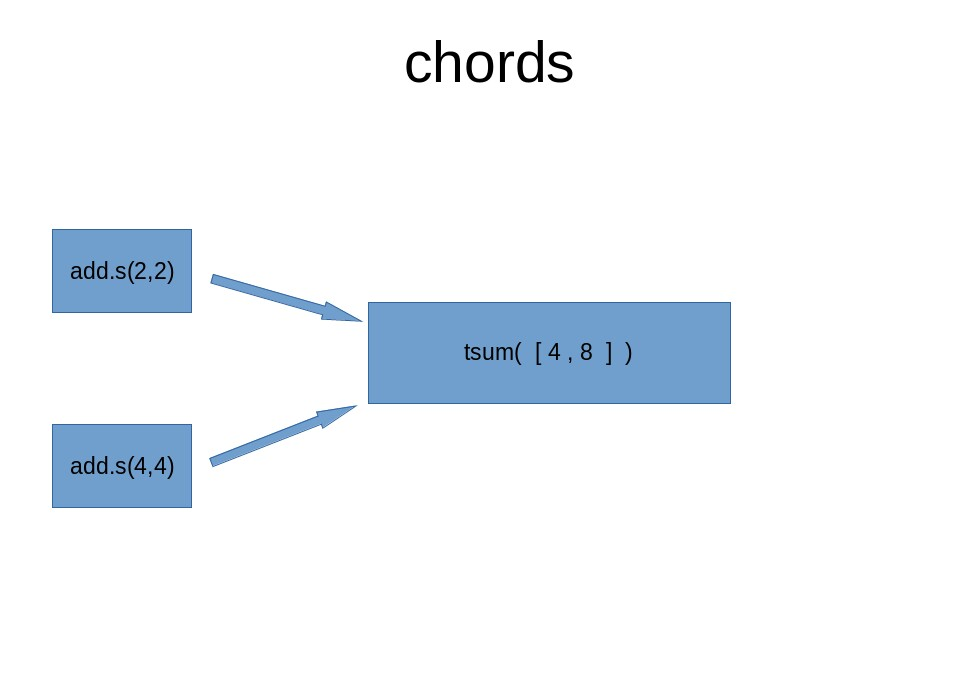## Chains, Groups and Chords in Celery

Feb. 6, 2021, 6:17 a.m.If you have used Celery with Django before then this tutorial will help you understand a few important concepts. If Celery is new to you , I suggest you check out their official documentation first just to get familiar with it.

# CHAINS

We are going to use this example task in our examples

``````@app.task
return x + y
``````

When we chain tasks together, the second task will take the results of the first task as its first argument, for example

``````

from celery import chain

``````

In the above example, you can notice the second task has only one argument , this is because the return value of the first task which in our example is 3 will be the first argument of the second task , the second task will now look like this

``````add.s(3, 3)
``````

Hope this image below helps you understand it betterNotice the first argument of second task is 3 and the first argument of third task is 6 which is the result of the second task.

Another way of chaining tasks is using this syntax with pipe symbol ( " | " ).

``````res2 = (add.s(1, 2) | add.s(3)).apply_async()
``````

The above code is just the same as this code below, you can choose which one you prefer.

`````` res2 = chain(add.s(1, 2), add.s(3)).apply_async()
``````

# GROUPS

Celery documentation says:

Groups are used to execute tasks in parallel. The group function takes in a list of signatures.

Example code :

``````

>>> from celery import group

>>> job = group([
... ])

>>> result = job.apply_async()

True
>>> result.successful() # were all subtasks successful?
True
>>> result.get()
[4, 8 ]

``````

Example image to illustrate how groups work# CHORDS

A Chord usually has two parts, header and callback

The syntax looks like this :

``````
``````

The header here is simply a group of tasks, the callback is run or executed after the groups of tasks have finished.

For us to demonstrate how chord works we will need a function which will act as our callback.

We will call our callback function tsum, it takes in a list of numbers and adds them up.

tsum:

``````@app.task
def tsum(numbers):
return sum(numbers)
``````

Chord example:

``````
>>> callback = tsum.s()
>>> result.get()
12

``````

The above code is similar to this mathematical expression:

∑ ((2 + 2) + (4 + 4))

Image below illustrates how chords workNote:

Celery documentation says we should avoid using chords as much as possible, but you can still use it if you really have to, read more on why you should not use chords on the Celery documentation.